Refer to our Texas Go Math Grade 5 Answer Key Pdf to score good marks in the exams. Test yourself by practicing the problems from Texas Go Math Grade 5 Lesson 1.2 Answer Key Thousandths.

Investigate

Materials: color pencils: straightedge

Thousandths are smaller parts than hundredths. If one hundredth is divided into ten equal parts, each part is one thousandth.
Use the model at the right to show tenths, hundredths, and thousandths.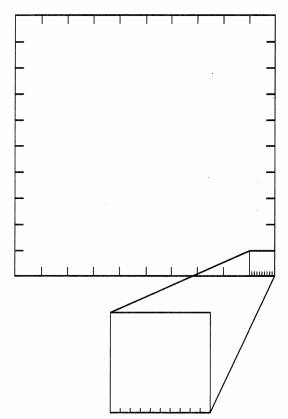A. Divide the larger square into 10 equal columns or rectangles. Shade one rectangle. What part of the whole is the shaded rectangle? Write that part as a decimal and a fraction.
It is given that
Divide the larger square into 10 equal columns or rectangles. Shade one rectangle
Now,
The representation of the larger square with one shaded rectangle is: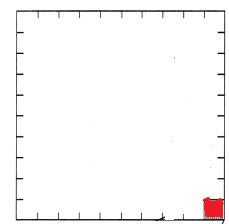Now,
From the given figure,
We can observe that
The shaded part is one-hundredth of the whole part
So,
The representation of one-hundredth as a fraction is: $$\frac{1}{100}$$
The representation of one-hundredth as a decimal is: 0.01
Hence, from the above,
We can conclude that
The shaded part is one-hundredth of the whole part
The representation of one-hundredth as a fraction is: $$\frac{1}{100}$$
The representation of one-hundredth as a decimal is: 0.01

B. Divide each rectangle into 10 equal squares. Use a second color to shade in one of the squares. What part of the whole is the shaded square? Write that part as a decimal and a fraction.
It is given that
Divide each rectangle into 10 equal squares. Use a second color to shade in one of the squares
Now,
The representation of the square that is divided into 10 squares with one shaded part is:Now,
From the given figure,
We can observe that
The shaded part of square is one-tenth of the whole square
So,
The representation of one-tenth as a fraction is: $$\frac{1}{10}$$
The representation of one-tenth as a decimal is: 0.1
Hence, from the above,
We can conclude that
The shaded part is one-hundredth of the whole part
The representation of one-tenth as a fraction is: $$\frac{1}{10}$$
The representation of one-tenth as a decimal is: 0.1

C. Divide the enlarged hundredths square into 10 equal columns or rectangles. If each hundredths square is divided into ten equal rectangles, how many parts will the model have?
It is given that
Divide the enlarged hundredths square into 10 equal columns or rectangles and each hundredths square is divided into ten equal rectangles
Now,
From the given information,
We can observe that
The total number of parts the model will have = $$\frac{100}{10}$$
= 10
Hence, from the above,
We can conclude that
The total number of parts the given model is: 10 parts

Use a third color to shade one rectangle of the enlarged hundredths square. What part of the whole is the shaded rectangle? Write that part as a decimal and a fraction.
It is given that
Use a third color to shade one rectangle of the enlarged hundredths square
Now,
The representation of the figure with the given information is: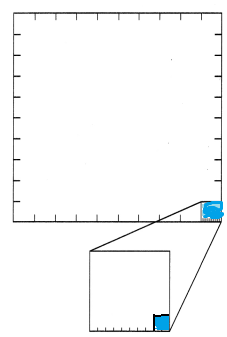Now,
From the given figure,
We can observe that
The whole part that is the shaded rectangle = $$\frac{1}{100}$$ × $$\frac{1}{10}$$
= $$\frac{1 × 1}{100 × 10}$$
= $$\frac{1}{1,000}$$
So,
The representation of the whole part that is the shaded rectangle in the form of a fraction is: $$\frac{1}{1,000}$$
The representation of the whole part that is the shaded rectangle in the form of a decimal number is: 0.001
Hence, from the above,
We can conclude that
The representation of the whole part that is the shaded rectangle in the form of a fraction is: $$\frac{1}{1,000}$$
The representation of the whole part that is the shaded rectangle in the form of a decimal number is: 0.001

Math Talk
Mathematical Processes
There are 10 times as many hundredths as there are tenths. Explain how the model shows this.
It is given that
There are 10 times as many hundredths as there are tenths
Hence, from the above,
Hence,
The representation of the model according to the given information is:Make Connections

The relationship of a digit in different place-value positions is the same with decimals as it is with whole numbers. You can use your understanding of place-value patterns and a place-value chart to write decimals that are 10 times as much as or $$\frac{1}{10}$$ of any given decimal.Use the steps below to complete the table.

STEP 1 Write the given decimal in a place-value chart.
STEP 2 Use the place-value chart to write a decimal that is 10 times as much as the given decimal.
STEP 3 Use the place-value chart to write a decimal that is $$\frac{1}{10}$$ 0f the given decimal.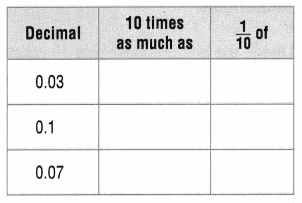Let the decimal number be 0.0x (or) 0.x
Now,
According to the given steps,
10 times of 0.0x is: 0.x
$$\frac{1}{10}$$ of 0.0x is: 0.00x
Now,
According to the given steps,
10 times of 0.x is: x
$$\frac{1}{10}$$ of 0.x is: 0.0x
So,
The completed table by using the above steps is: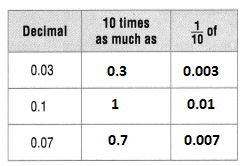Hence, from the above,
We can conclude that
The completed table is:Math Talk
Mathematical Processes

Describe the pattern you see when you move one decimal place value to the right and one decimal place value to the left.
Let the decimal number be 0.0x (or) 0.x
Now,
When you move one decimal place to the right,
The value of 0.0x will be: 0.00x
The value of 0.x will be: 0.0x
Now,
When you move one decimal place to the left,
The value of 0.0x will be: 0.x
The value of 0.x will be: x
Hence, from the above,
We can conclude that
a.
When you move one decimal place to the right,
The value of 0.0x will be: 0.00x
The value of 0.x will be: 0.0x
b.
When you move one decimal place to the left,
The value of 0.0x will be: 0.x
The value of 0.x will be: x

Share and Show

Question 1.
Write the decimal shown by the shaded part of the model.The given figure is:Now,
From the given figure,
We can observe that
The total number of parts are: 100
The number of shaded parts is: 1
So,
The representation of the shaded part from the whole part is: $$\frac{1}{100}$$
Now,
The representation of the shaded part from the whole part in the form of a decimal number is: 0.01
Hence, from the above,
We can conclude that
The representation of the shaded part from the whole part in the form of a decimal number is: 0.01

Use place-value patterns to complete the table.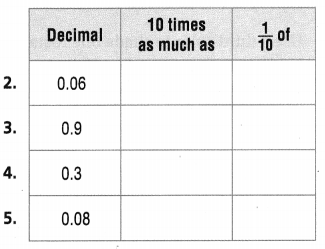Let the decimal number be 0.0x (or) 0.x
Now,
10 times of 0.0x is: 0.x
$$\frac{1}{10}$$ of 0.0x is: 0.00x
Now,
10 times of 0.x is: x
$$\frac{1}{10}$$ of 0.x is: 0.0x
Hence,
The completed table by using the place-value patterns is: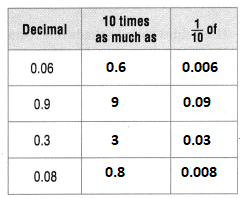Problem Solving

Use the table for 6-9.Question 6.
What is the value of the digit 2 in the carpenter bee’s length?
The given table is: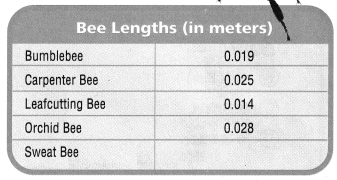Now,
From the given table,
We can observe that
The length of the carpenter Bee is: 0.025 m
Now,
The representation of the place-value table for the length of the carpenter Bee is:So,
From the given table,
We can observe that
The value of the digit 2 is: 0.02
Hence, from the above,
We can conclude that
The value of the digit 2 in the Carpenter Bee’s length is: 0.02

Question 7.
If you made a model of a bumblebee that was 10 times as large as the actual bee, how long would the model be in meters? Write your answer as a decimal.
It is given that
you made a model of a bumblebee that was 10 times as large as the actual bee
Now,
The given table is:Now,
From the given table,
We can observe that
The length of the Bumblebee is: 0.019 meters
So,
According to the given information,
(The length of the bumble bee) × 10 = The length of the model of bumblebee
= 0.019 × 10
= 0.19 meters
Hence, from the above,
We can conclude that
The length of the model of Bumble bee is: 0.19 meters

Question 8.
Record The sweat bee’s length is 6 thousandths of a meter. Complete the table by recording the sweat bee’s length.
It is given that
The sweat bee’s length is 6 thousandths of a meter
Now,
The given table is:Now,
According to the given information,
The length of the sweat bee is: 0.006 meters
Now,
The representation of the length of sweat bee in the place value table is: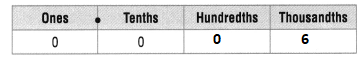Hence, from the above,
We can conclude that
The completed table with sweat bee’s length is:Question 9.
H.O.T. An atlas beetle is about 0.14 of a meter long. How does the length of the atlas beetle compare to the length of a leaf cutting bee?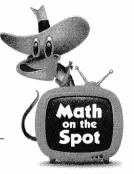It is given that
An atlas beetle is about 0.14 of a meter long
Now,
The given table is:Now,
From the given table,
We can observe that
The length of the Leaf cutting Bee is: 0.014 meters
Now,
According to the given information,
The length of an Atlas Bee is 10 times as long as the length of the Leaf cutting bee
Hence, from the above,
We can conclude that
The length of an Atlas Bee is 10 times as long as the length of the Leaf cutting bee

Question 10.
H.O.T. Multi-Step Terry, Sasha, and Harry each chose a number. Sasha’s number is 10 times as much as Harry’s number. Terry’s number is 10 times as much as Sasha’s number. Harry’s number is 0.075. What number did each person choose?
It is given that
Terry, Sasha, and Harry each chose a number. Sasha’s number is 10 times as much as Harry’s number. Terry’s number is 10 times as much as Sasha’s number. Harry’s number is 0.075
Now,
According to the given information,
Sasha’s number = 10 × Harry’s number
Terry’s number = 10 × Sasha’s number
So,
Sasha’s number is: 0.75
Terry’s number is: 7.5
Hence, from the above,
We can conclude that
Sasha’s number is: 0.75
Terry’s number is: 7.5

Question 11.
Write Math Explain how you can use place value to describe how 0.05 and 0.005 compare.
The given decimal numbers are: 0.05 and 0.005
Now,
The representation of the given decimal numbers in the place-value table is: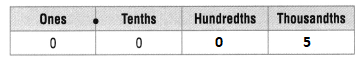Now,
From the given place-values tables,
We can observe that
The hundredth’s place in 0.05 is greater than the hundredth’s place in 0.005
Hence, from the above,
We can conclude that
0.05 is greater than 0.005

Question 12.
A squirrel is 1.1 feet tall. The squirrel shrinks to $$\frac{1}{10}$$ of its height. How tall is the squirrel now?
A. 11.0 feet
B. 0.11 feet
C. 1.10 feet
D. 0.011 feet
It is given that
A squirrel is 1.1 feet tall. The squirrel shrinks to $$\frac{1}{10}$$ of its height
So,
The present height of squirrel = 1.1 × $$\frac{1}{10}$$
= $$\frac{11}{10}$$ × $$\frac{1}{10}$$
= $$\frac{11 × 1}{10 ×10}$$
= 0.11 feet
Hence, from the above,
We can conclude that
The height of the squirrel now is: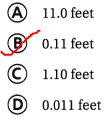Question 13.
Use Diagrams Write the decimal shown by the model.The given figure is:Now,
From the given figure,
We can observe that
From the bigger rectangle,
The part of the shaded from the whole part = (The number of shaded parts) ÷ (The total number of parts)
= $$\frac{18}{100}$$
= 0.18
Now,
From the square,
The part of the shaded from the whole part = (The 1 part of the shaded part in the rectangle) × (The part of te shaded part of the square)
= $$\frac{1}{100}$$ × $$\frac{1}{10}$$
= $$\frac{1}{1,000}$$
= 0.001
So,
The total shaded part fro the given figure = 0.18 + 0.001
= 0.181
Hence, from the above,
We can conclude that
The decimal number that is shown by the given model is:Question 14.
Multi-Step A chef uses 0.03 liter olive oil to make soup. He uses 10 times as much chicken broth as olive oil. He uses 10 times as much vegetable broth as chicken broth. How much vegetable broth does he use?
A. 0.003 liter
B. 0.03 liter
C. 0.3 liter
D. 3 liters
It is given that
A chef uses 0.03 liter olive oil to make soup. He uses 10 times as much chicken broth as olive oil. He uses 10 times as much vegetable broth as chicken broth
So,
The amount of chicken broth a chef used = 10 × (The amount of olive oil used by a chef)
The amount of vegetable broth a chef used = 10 × (The amount of chicken broth a chef used)
So,
The amount of chicken broth a chef used = 10 ×0.03
= 0.3 liters
The amount of vegetable broth a chef used = 10 × 0.3
= 3 liters
Hence, from the above,
We can conclude that
The amount of vegetable broth the chef used is: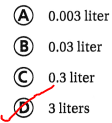Texas Test Prep

Question 15.
What is the relationship between 1.0 and 0.1?
A. 0.1 is 10 times as much as 1.0
B. 1.0 is $$\frac{1}{10}$$ of 0.1
C. 0.1 is $$\frac{1}{10}$$ of 1.0
D. 1.0 is equal to 0.1
The given decimal numbers are: 1.0 and 0.1
Now,
1.0 is 10 times as 0.1
0.1 is $$\frac{1}{10}$$ of 1
Hence, from the above,
We can conclude that
The relationship between 1.0 and 0.1 is:### Texas Go Math Grade 5 Lesson 1.2 Homework and Practice Answer Key

Write the decimal shown by the shaded part of the model.

Question 1.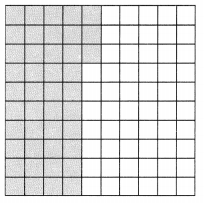The given figure is:Now,
From the given figure,
We can observe that
The total number of parts are: 100
The number of shaded parts are: 33
So,
The part of the shaded from the whole part = (The number of shaded parts) ÷ (The total number of parts)
= $$\frac{33}{100}$$
= 0.33
Hence, from the above,
We can conclude that
The decimal shown by the shaded part is: 0.33

Question 2.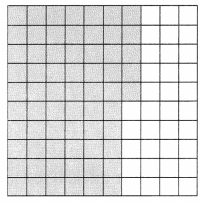The given figure is:Now,
From the given figure,
We can observe that
The total number of parts are: 100
The number of shaded parts are: 75
So,
The part of the shaded from the whole part = (The number of shaded parts) ÷ (The total number of parts)
= $$\frac{75}{100}$$
= 0.75
Hence, from the above,
We can conclude that
The decimal shown by the shaded part is: 0.75

Use place-value patterns to complete the table.

Question 3.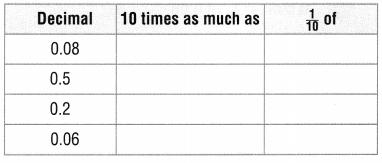Let the decimal number be 0.0x (or) 0.x
Now,
10 times of 0.0x is: 0.x
$$\frac{1}{10}$$ of 0.0x is: 0.00x
Now,
10 times of 0.x is: x
$$\frac{1}{10}$$ of 0.x is: 0.0x
Hence,
The completed table by using the place-value patterns is:Question 4.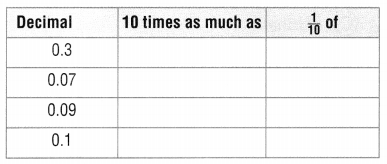Let the decimal number be 0.0x (or) 0.x
Now,
10 times of 0.0x is: 0.x
$$\frac{1}{10}$$ of 0.0x is: 0.00x
Now,
10 times of 0.x is: x
$$\frac{1}{10}$$ of 0.x is: 0.0x
Hence,
The completed table by using the place-value patterns is: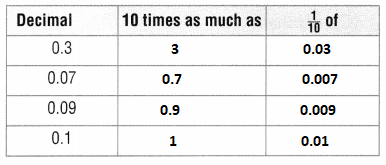Problem Solving

Question 5.
Andy makes a model of a new tool that is 0.24 meter long. His model is 10 times as large as the actual tool. How big is the model?
It is given that
Andy makes a model of a new tool that is 0.24 meter long. His model is 10 times as large as the actual tool
So,
The length of the model = 10 × (The length of the actual model)
= 10 × 0.24
= 2.4 meters
Hence, from the above,
We can conclude that
The length of the model is: 2.4 meters

Question 6.
Jamie makes a necklace with beads that are 0.08 meter long. Her next necklace will have beads that are $$\frac{1}{10}$$ the size of the beads of the first necklace. What will be the length of the beads for the second necklace?
It is given that
Jamie makes a necklace with beads that are 0.08 meter long. Her next necklace will have beads that are $$\frac{1}{10}$$ the size of the beads of the first necklace
So,
The length of the beads for the second necklace = $$\frac{1}{10}$$ × (The length of the beads for the first necklace)
= $$\frac{1}{10}$$ × 0.08
= 0.008 meters
Hence, from the above,
We can conclude that
The length of the beads for the second necklace is: 0.008 meters

Lesson Check

Question 7.
What is the value of the digit 1 in the number 0.413?
A. 1 tenth
B. 1 hundredth
C. 1 hundreds
D. 1 tens
The given decimal number is: 0.413
So,
The representation of the given number in the place-value table is: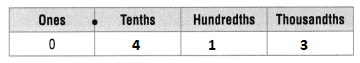Hence, from the above,
We can conclude that
The value of the digit 1 in the given number is: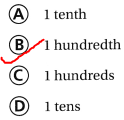Question 8.
How does 0.03 compare to 0.003?
A. 0.03 is 10 times as much as 0.003
B. 0.03 is 100 times as much as 0.003
C. 0.03 is $$\frac{1}{10}$$ of 0.003
D. 0.03 is $$\frac{1}{100}$$ of 0.003
The given decimal numbers are: 0.03 and 0.003
Now,
From the given numbers,
We can observe that
0.03 is $$\frac{1}{10}$$ of 0.003
Hence, from the above,
We can conclude that
The relationship between 0.03 and 0.003 is:Question 9.
The ice on a pond is 0.03 meter thick. If the ice were 10 times as thick, how many meters thick would the ice be?
A. 0.003 meter
B. 0.3 meter
C. 3 meters
D. 3.03 meters
It is given that
The ice on a pond is 0.03 meter thick
So,
The new thickness of the ice = 10 × (The thickness of old ice on the pond)
= 10 × 0.03
= 0.3 meters
Hence, from the above,
We can conclude that
The thickness of the new ice is: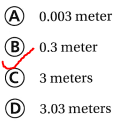Question 10.
How does 0.008 compare to 0.08?
A. 0.008 is 10 times as much as 0.08
B. 0.008 is 100 times as much as 0.08
C. 0.008 is $$\frac{1}{10}$$ of 0.08
D. 0.008 is $$\frac{1}{100}$$ of 0.08
The given decimal numbers are: 0.008 and 0.08
Now,
From the given numbers,
We can observe that
0.008 is $$\frac{1}{10}$$ of 0.08
Hence, from the above,
We can conclude that
The relationship between 0.008 and 0.08 is: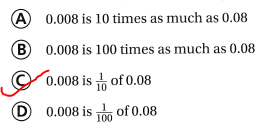Question 11.
Multi-Step A green ribbon is 9 inches long. A red ribbon is $$\frac{1}{10}$$ the length of the green ribbon. A purple ribbon is 10 times as long as the red ribbon. What is the length of the purple ribbon?
A. 0.09 inch
B. 9 inches
C. 0.9 inch
D. 90 inches
It is given that
A green ribbon is 9 inches long. A red ribbon is $$\frac{1}{10}$$ the length of the green ribbon. A purple ribbon is 10 times as long as the red ribbon
So,
The length of the red ribbon = $$\frac{1}{10}$$ × (The length of the green ribbon)
= $$× 9 = 0.9 meters So, The length of the purple ribbon = 10 × (The length of the red ribbon) = 10 ×0.9 = 9 meters Hence, from the above, We can conclude that The length of the purple ribbon is: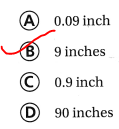Question 12. Multi-Step Alan lives 10 times as far from school as Linda. Linda lives 0.7 mile from school. Jenny lives [latex]\frac{1}{10}$$ as far from school as Alan. Pedro lives $$\frac{1}{10}$$ as far from school as Jenny. How far from school does Pedro live?
A. 7 miles
B. 0.07 mile
C. 70 miles
D. 0.7 mile
It is given that
Alan lives 10 times as far from school as Linda. Linda lives 0.7 mile from school. Jenny lives $$\frac{1}{10}$$ as far from school as Alan. Pedro lives $$\frac{1}{10}$$ as far from school as Jenny
So,
The distance from school to Alan’s house = 10 × (The distance from the school to Linda’s house)
= 10 × 0.7
= 7 miles
So,
The distance from school to Jenny’s house = $$\frac{1}{10}$$ × (The distance from the school to Alan’s house)
= $$\frac{1}{10}$$ × 7
= 0.7 miles
The distance from school to Pedro’s house = $$\frac{1}{10}$$ × (The distance from the school to Jenny’s house)
= $$\frac{1}{10}$$ × 0.7
= 0.07 miles
Hence, from the above,
We can conclude that
The distance from school to Pedro’s house is:Scroll to Top